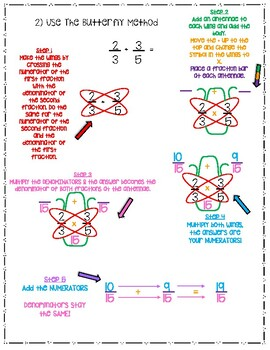# Fractions Anchor Charts (Equivalent and +/-) CCSS: 4.NF.A.2 & 5.NF.A.1Subject
Resource Type
File Type

PDF

(413 KB|5 pages)
Product Rating
Standards
• Product Description
• StandardsNEW

Equivalent Fractions & Adding/Subtracting Fractions with Unlike Denominators Anchor Charts

CCSS: 4.NF.A.2 & 5.NF.A.1

Equivalent Fractions

1) Multiplying numerators & denominators by the same number

2) Skip Counting

3) Cross Multiplying to check equivalence

1) Using a T-chart

2) Butterfly method

Add and subtract fractions with unlike denominators (including mixed numbers) by replacing given fractions with equivalent fractions in such a way as to produce an equivalent sum or difference of fractions with like denominators. For example, 2/3 + 5/4 = 8/12 + 15/12 = 23/12. (In general, 𝘢/𝘣 + 𝘤/𝘥 = (𝘢𝘥 + 𝘣𝘤)/𝘣𝘥.)
Compare two fractions with different numerators and different denominators, e.g., by creating common denominators or numerators, or by comparing to a benchmark fraction such as 1/2. Recognize that comparisons are valid only when the two fractions refer to the same whole. Record the results of comparisons with symbols >, =, or <, and justify the conclusions, e.g., by using a visual fraction model.
Total Pages
5 pages
Does not apply
Teaching Duration
N/A
Report this Resource to TpT
Reported resources will be reviewed by our team. Report this resource to let us know if this resource violates TpT’s content guidelines.